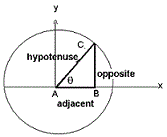# Trigonometry Functions Calculation

Calculate the values for six possible trigonometric functions or ratios as sine, cosine, tangent, cotangent, secant and cosecant against selection, using following formulas:Sinθ = 1 / Cosecθ
Cosθ = 1 / Secθ
Tanθ = Sinθ / Cosθ
Cosecθ = 1 / Sinθ
Secθ = 1 / Cosθ
Cotθ = 1 / Tanθ
Trigonometry Value:
 Sin(x): Cos(x): Tan(x): cosec(x): Sec(x): Cot(x):
The trigonometric functions are functions of an angle and side measurement. It is relate the angles of a triangle to the lengths of its sides.
The summarized table for trigonometric functions and important Formula as follows: